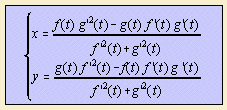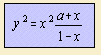# pedal curve

## mainGiven a curve C1 and a (pedal) point O, construct for each tangent l of C1 a point P, for which OP is perpendicular to the tangent. The collection of points P forms a curve C2, the (positive) pedal of C1 (with respect to the pedal point).
When C1 is given by (x, y) = (f(t), g(t)), and we translate C1 in such a way that the pedal point is the origin, then C2 has the form:Two curves are invariant for making a pedal:

The pedal of the parabola is the curve given by the equation:a pedal point pedal of the parabola 0 vertex cissoid (of Diocles) 1 foot of - intersection of axis and - directrix (right) strophoid 3 reflection of focus in directrix trisectrix of MacLaurin - on directrix oblique strophoid - focus line

Some other pedals are:

 curve pedal point pedal astroid center quadrifolium cardioid cusp Cayley's sextic circle any point lima�on on circle cardioid cissoid (of Diocles) focus cardioid deltoid cusp simple folium vertex regular bifolium center trifolium on the curve bifolium ellipse focus circle epicycloid center rhodonea equiangular spiral pole equiangular spiral hyperbola focus circle rectangular hyperbola center lemniscate hypocycloid center rhodonea involute of a circle center Archimedes' spiral line any point point parabola focus line on directrix strophoid center of directrix right strophoid reflection of focus by directrix trisectrix of Maclaurin vertex cissoid sinusoidal spiral pole sinusoidal spiral Talbot's curve center ellipse Tschirnhausen's cubic focus parabola

The reverse operation of making a pedal is to construct from each point P of C2 a line l that is perpendicular to OP. The lines l together form an envelope of the curve C1. Now we call C1 the negative pedal 1) of C2. When C1 is a pedal of C2, then C2 is the negative pedal of C1.
Because of this definition, the curve is in fact also an orthocaustic: the orthocaustic of a curve C1 (with respect to a point O) is the envelope of the perpendiculars of P on OP (P on C1).

Instead of tangents to a curve we can consider normals to that curve. This pedal curve is called the normal pedal curve.

MacLaurin was the first author to investigate pedal curves (1718).

notes

1) In French: antipodaire. In German: Gegenfusspunktskurve.